Next: Fitting Parameterized Models Up: Curve Fitting Previous: Fitting Straight Lines

### Fitting General Curves

The line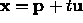is a special case of the general parametric curve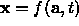, where t moves x along the curve defined by parameters a. Finding the parameters, a, which best fit such a general curve through a set of 2D data points can be complicated because of the difficulty of defining and evaluating a suitable likelihood function. Simply calculating the closest approach distance of the curve to a single data point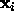requires a minimization in itself (ie Find t which minimizes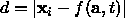). This leads to nasty nested minimization problems of the form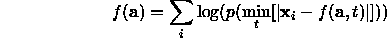A special case worth describing is when the curve can be written in the form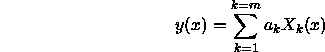where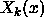are arbitrary fixed functions of x called basis functions. The only unknown parameters are the m weights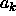. If we wish to find the best fit of such a function to N points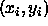where the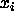are known precisely but the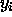have a gaussian measurement error with standard deviation of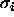, we can use and approach called General Least Squares. We define a log-likelihood function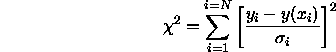The parameters a which minimize this can be found as follows. Define an N x m design matrix D such that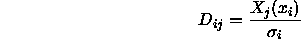Define an N element vector r such that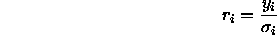It can be shown  that the optimal choice of parameters is given by using a singular value decomposition to give the least squares solution to the linear equation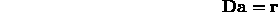If D is decomposed as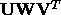with V m x m and orthonormal, then the covariance of the estimate of parameters a is given by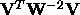.

Bob Fisher
Fri Mar 28 14:12:50 GMT 1997